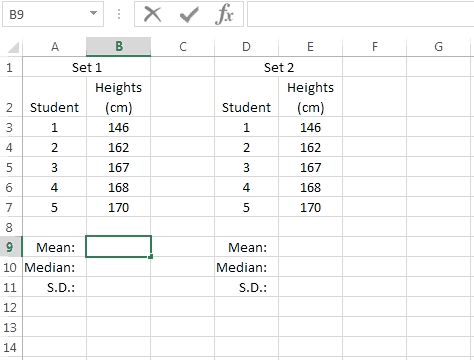### Excel formula

#### Summary Statistics - syllabus example | # gomaths.net/4238         User submitted, thanks to Simon Job

Use a spreadsheet to examine the effect on the calculate summary statistics of changing the value of a score.

Syllabus: Mathematics General Course, page 37

Mean: `=AVERAGE(B3:B7)`

Median: `=MEDIAN(B3:B7)`

S.D.: `=STDEV.P(B3:B7)`

where `B3:B7` is the range of cells containing numerical data.